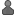# New Math Formula

 Keywords Advanced search

Message boards : MilkyWay@home Science : New Math Formula
Message board moderation

 To post messages, you must log in. "Oldest first Newest first Highest rated posts first

AuthorMessageScientific Frontline

Joined: 21 Jun 08
Posts: 4
Credit: 2,710
RAC: 0Message 4252 - Posted: 19 Jul 2008, 20:42:33 UTC

I do like the new longer WU's
But I am not familiar with the math used to calculate.
I just received 18 units at an Avg. completion rate of 6hr. each, that is fine, but due in one day... Now I am looking for a way to do 118 hrs work in 24, but can't get the Delorean started. ~smiles~
Heidi-Ann Kennedy
Scientific FrontlineNathan
Project scientistJoined: 4 Oct 07
Posts: 43
Credit: 53,898
RAC: 0Message 4420 - Posted: 23 Jul 2008, 15:50:10 UTC
Last modified: 23 Jul 2008, 15:50:49 UTC

Well you just need to get your flux capacitor calibrated.

Yeah, there are some timings that need ironed out with WU number and deadlines, we're working on that.

As for the math used to calculate the WU work: the length of the WUs are almost entirely determined by a numerical integral within the code. Basically what happens is that we take the volume that we are studying and subdivide it into many smaller volumes and calculate what the probabilities are for the spheroid and the stream within each sub-volume and add them up to get a total integral value for the big volume. The number of subdivisions that we use effectively controls the run-time of the algorithm. The actual math is determined by the models of our stream and spheroid (which can be found in the paper here) and the shape of the volume we are looking at (in this case a wedge, so we create a number of small wedges in which the volume can be calculated using trigonometry). We multiply the subvolumes by probabilities and sum all these values to get our total integral.
~Nate~

Message boards : MilkyWay@home Science : New Math Formula

©2019 Astroinformatics Group